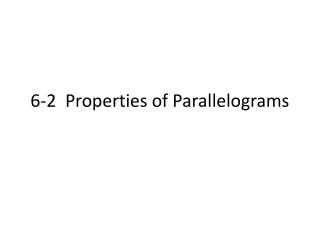DownloadDownload Presentation6-2 Properties of Parallelograms

# 6-2 Properties of Parallelograms

Download Presentation## 6-2 Properties of Parallelograms

- - - - - - - - - - - - - - - - - - - - - - - - - - - E N D - - - - - - - - - - - - - - - - - - - - - - - - - - -
##### Presentation Transcript

1. 6-2 Properties of Parallelograms

2. Quadrilaterals • In a quadrilateral, opposite sides do not share a vertex and opposite angles do not share a side. • In other words, they are ACROSS from each other. • Angles of a polygon that share a side are consecutive angles.

3. Parallelograms • A parallelogram is a quadrilateral with both pairs of opposite sides parallel. Theorem 6-3: If a quadrilateral is a parallelogram, then its opposite sides are congruent. Theorem 6-4: If a quadrilateral is a parallelogram, then its consecutive angles are supplementary. Theorem 6-5: If a quadrilateral is a parallelogram, then its opposite angles are congruent. Theorem 6-6: If a quadrilateral is a parallelogram, then its diagonals bisect each other.

4. Using Consecutive Angles • What is mP?  Suppose you adjust the lamp so thatmS = 86. What is mR?

5. Using Algebra to Find Lengths • Solve a system of linear equations to find the values of x and y. What are KM and LN?

6.  Find the values of x and y. What are PR and SQ?

7. Parallel Lines and Transversals Theorem 6-7: If three (or more) parallel lines cut off congruent segments on one transversal, then they cut off congruent segments on every transversal.

8. Using Parallel Lines and Transversals • In the figure, marked lines are parallel, AB = BC = CD = 2, and EF = 2.25. What is EH?  If EF = FG = GH = 6 and AD = 15, what is CD?

9. 6-3 Proving That a Quadrilateral Is a Parallelogram